# RD Sharma Solutions for Class 9 Maths Chapter 23 Graphical Representation of Statistical Data Exercise 23.3

RD Sharma Solutions for Class 9th Graphical Representation of Statistical Data will help you to understand the steps involved in representing a large amount of statistical data in graphs from the very beginning. In this exercise, of RD Sharma Solutions class 9 students will learn about construction of histogram of a continuous grouped frequency distribution with unequal class intervals and many more.

## Download PDF of RD Sharma Solutions for Class 9 Maths Chapter 23 Graphical Representation of Statistical Data Exercise 23.3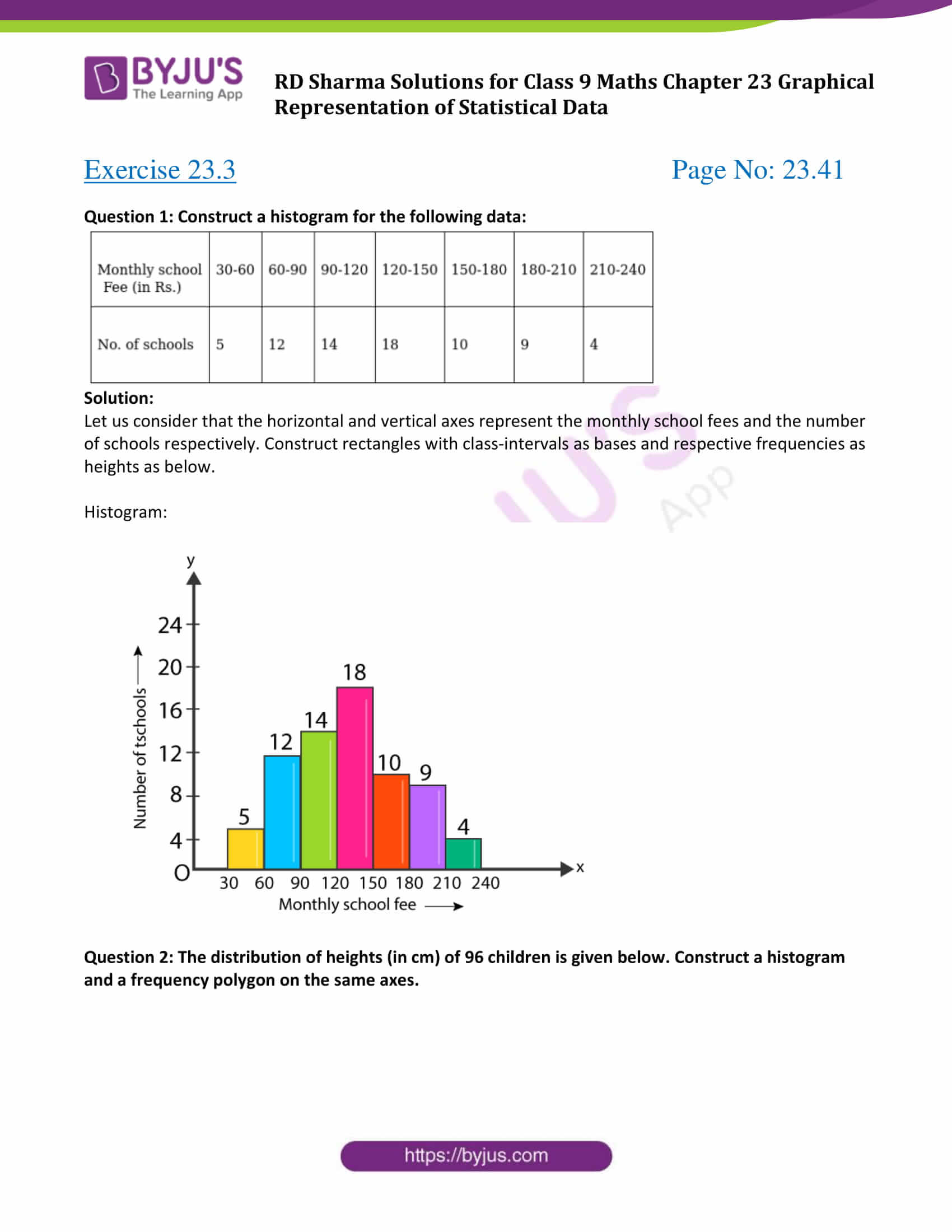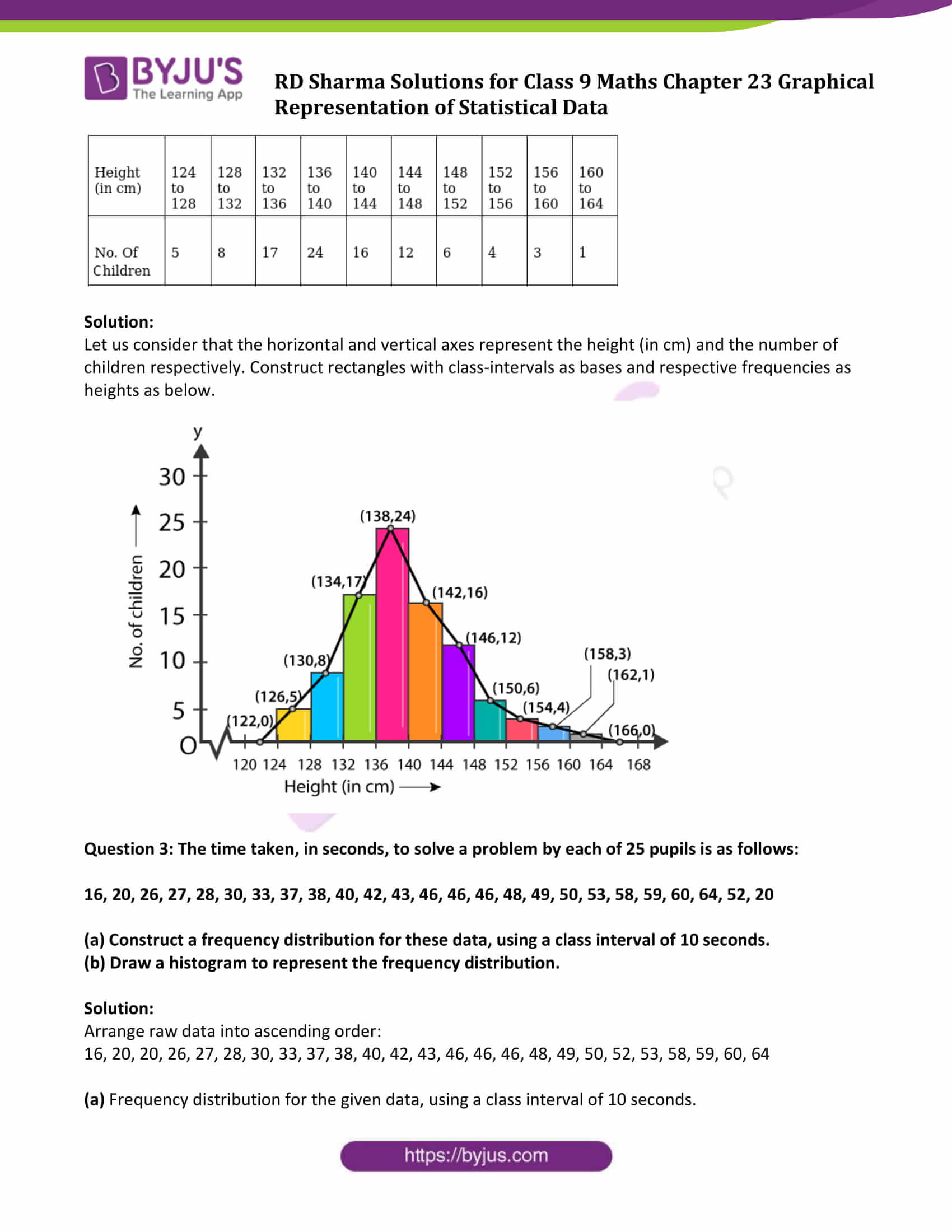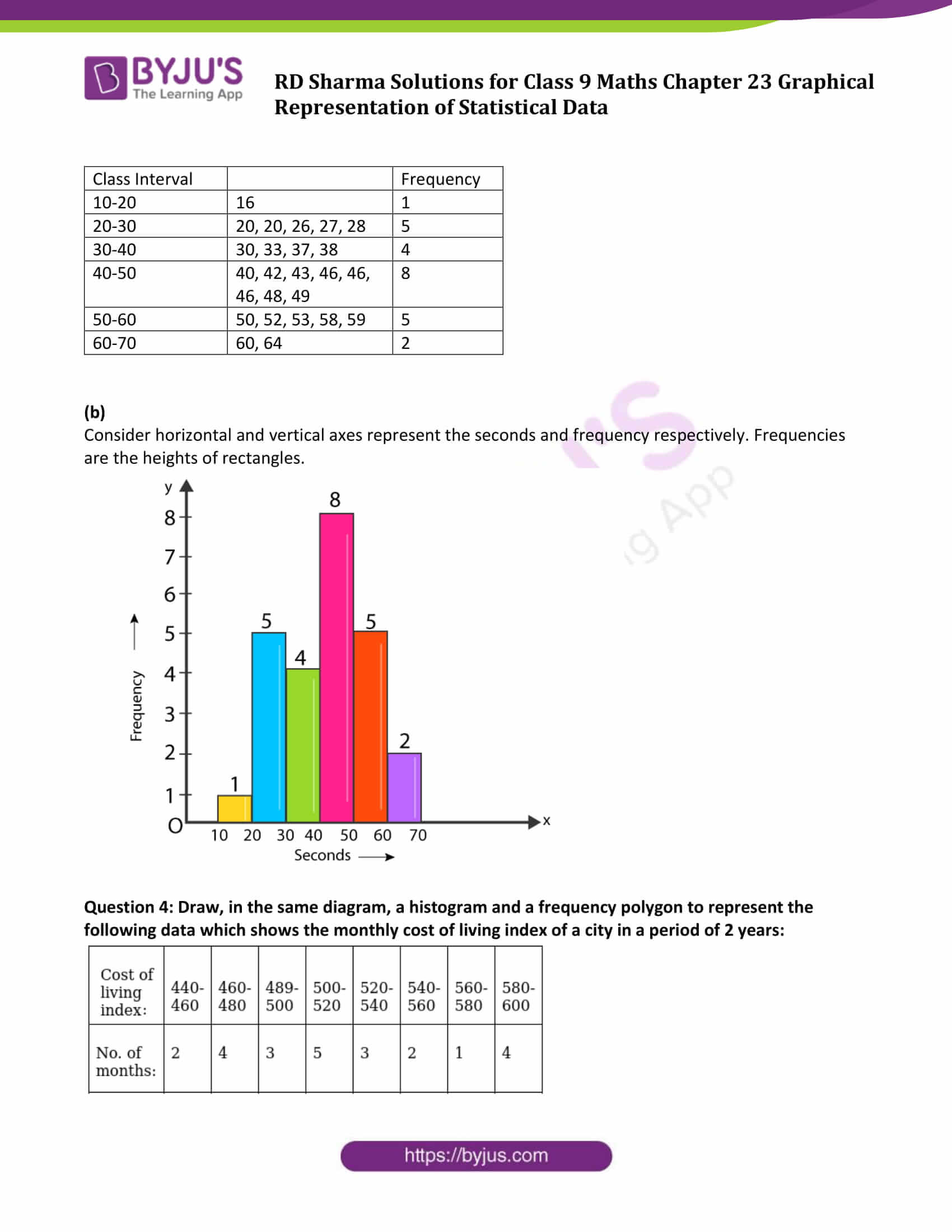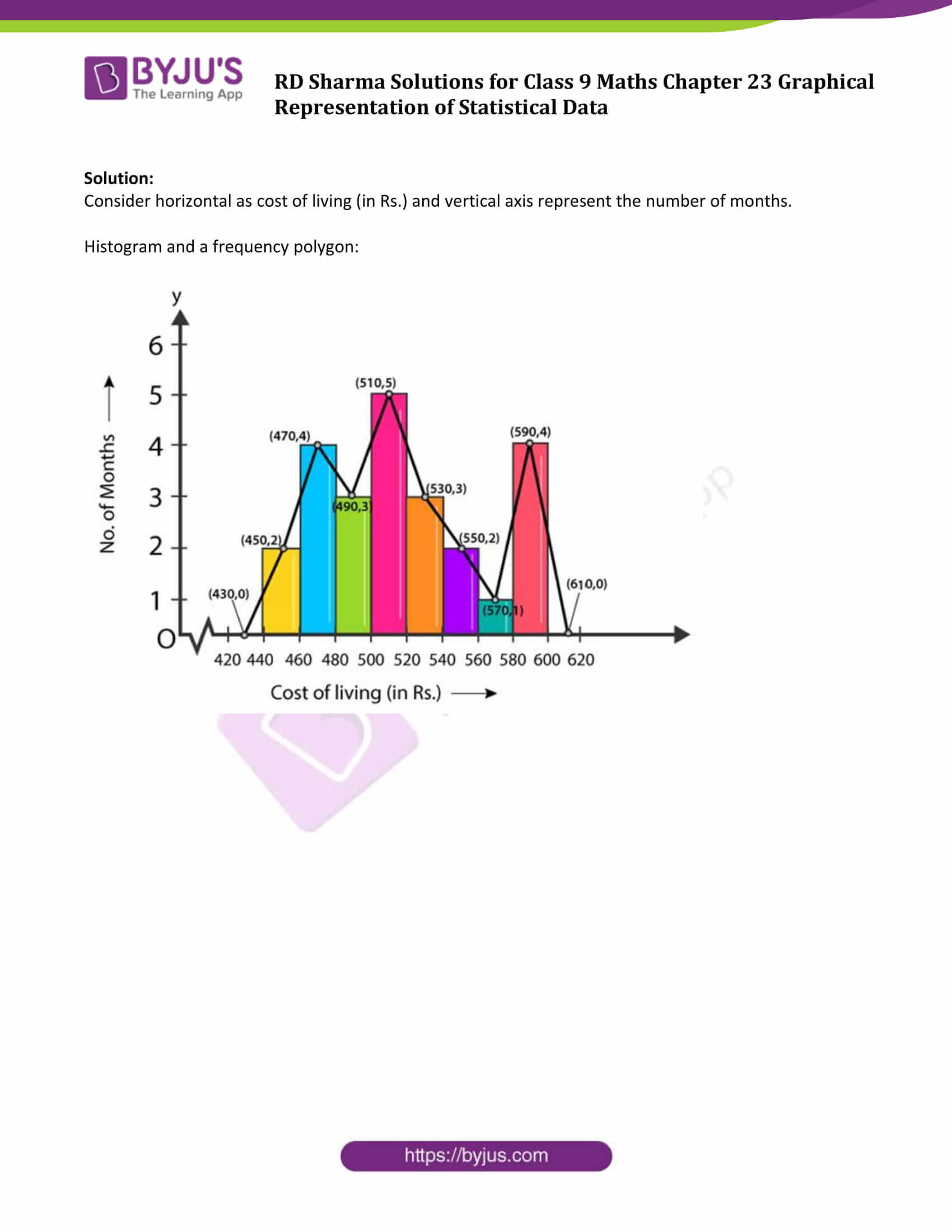### Access Answers to Maths RD Sharma Solutions for Class 9 Chapter 23 Graphical Representation of Statistical Data Exercise 23.3 Page number 23.41

Question 1: Construct a histogram for the following data: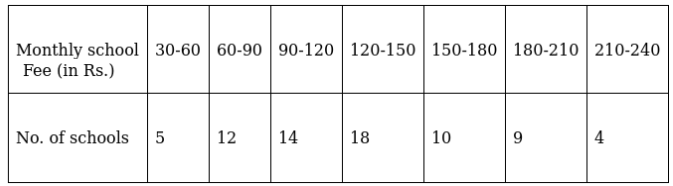Solution:

Let us consider that the horizontal and vertical axes represent the monthly school fees and the number of schools respectively. Construct rectangles with class-intervals as bases and respective frequencies as heights as below.

Histogram: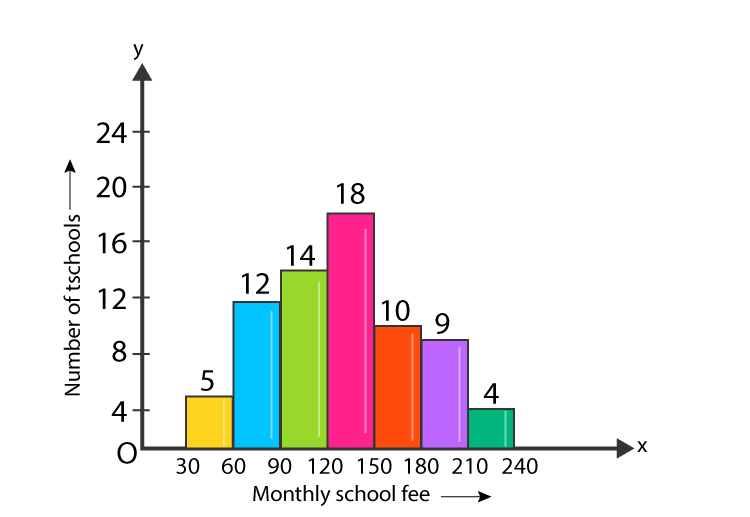Question 2: The distribution of heights (in cm) of 96 children is given below. Construct a histogram and a frequency polygon on the same axes.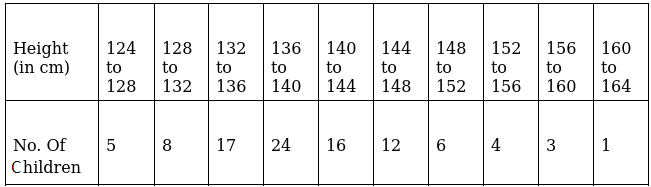Solution:

Let us consider that the horizontal and vertical axes represent the height (in cm) and the number of children respectively. Construct rectangles with class-intervals as bases and respective frequencies as heights as below.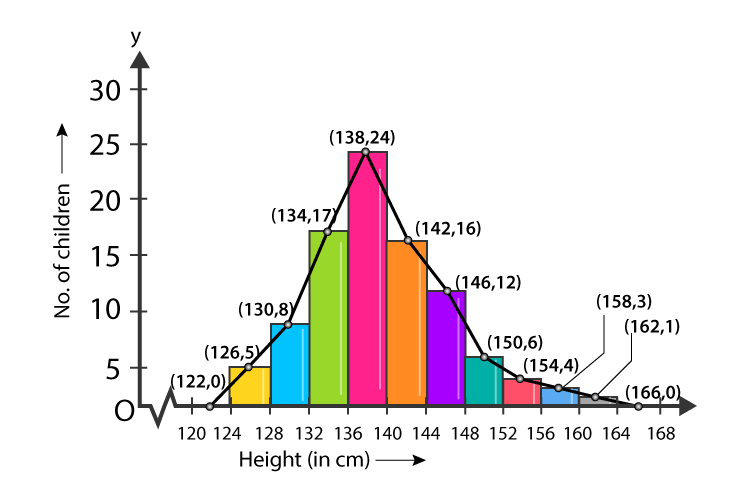Question 3: The time taken, in seconds, to solve a problem by each of 25 pupils is as follows:

16, 20, 26, 27, 28, 30, 33, 37, 38, 40, 42, 43, 46, 46, 46, 48, 49, 50, 53, 58, 59, 60, 64, 52, 20

(a) Construct a frequency distribution for these data, using a class interval of 10 seconds.

(b) Draw a histogram to represent the frequency distribution.

Solution:

Arrange raw data into ascending order:

16, 20, 20, 26, 27, 28, 30, 33, 37, 38, 40, 42, 43, 46, 46, 46, 48, 49, 50, 52, 53, 58, 59, 60, 64

(a) Frequency distribution for the given data, using a class interval of 10 seconds.

 Class Interval Frequency 10-20 16 1 20-30 20, 20, 26, 27, 28 5 30-40 30, 33, 37, 38 4 40-50 40, 42, 43, 46, 46, 46, 48, 49 8 50-60 50, 52, 53, 58, 59 5 60-70 60, 64 2

(b)

Consider horizontal and vertical axes represent the seconds and frequency respectively. Frequencies are the heights of rectangles.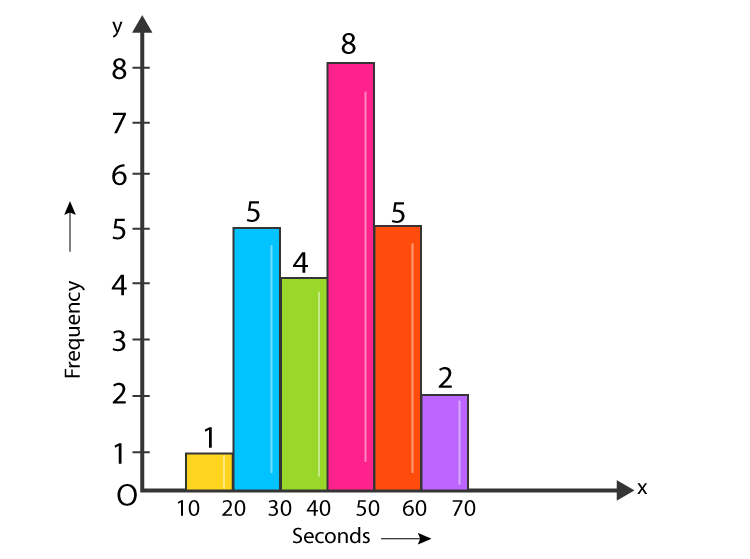Question 4: Draw, in the same diagram, a histogram and a frequency polygon to represent the following data which shows the monthly cost of living index of a city in a period of 2 years: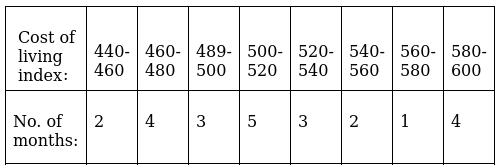Solution:

Consider horizontal as cost of living (in Rs.) and vertical axis represent the number of months.

Histogram and a frequency polygon: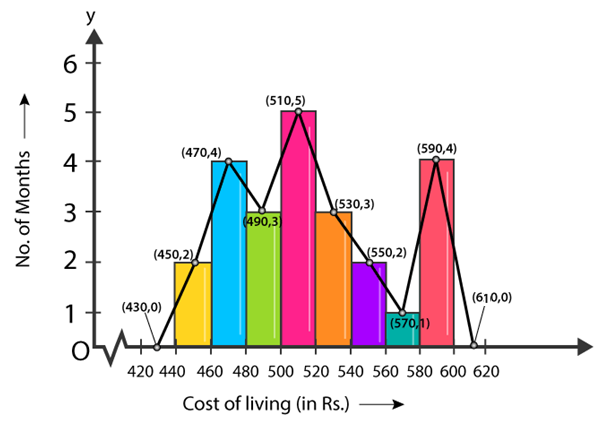## RD Sharma Solutions for Class 9 Maths Chapter 23 Graphical Representation of Statistical Data Exercise 23.3

RD Sharma Solutions Class 9 Maths Chapter 23 Graphical Representation of Statistical Data Exercise 23.3 is based on the following topics:

• Histogram
• Construction of a histogram of a continuous grouped frequency distribution with unequal class intervals.
• Construction of a histogram when mid-points of class intervals are given.
• Construction of a histogram when class intervals are given in inclusive form
• Frequency polygon and its algorithm